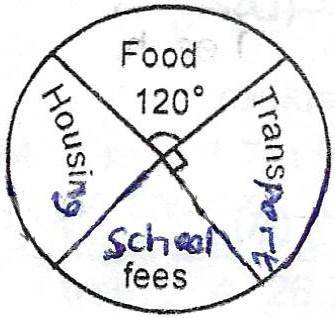### Mathematics 2018 JAMB Past Questions

1

In a class of 40 students, 32 offer Mathematics, 24 offer Physics and 4 offer neither Mathematics nor Physics. How many offer both Mathematics and Physics?

• A. 4
• B. 8
• C. 16
• D. 20
JAMB 2018
2

Find the values of x for which

$$\frac {x+2}{4}$$ - $$\frac{2x - 3}{3}$$ < 4

• A. x < 8
• B. x > -6
• C. x < 4
• D. x > -3
JAMB 2018
3The pie chart shows the monthly expenditure of a public servant. The monthly expenditure on housing is twice that of school fees. How much does the worker spend on housing if his monthly income is N7200?

• A. 1000
• B. 2000
• C. 3000
• D. 4000
JAMB 2018
4

A trader realises 10x - x$$^2$$ Naira profit from the sale of x bags of corn. How many bags will give him the maximum profit?

• A. 7
• B. 6
• C. 5
• D. 4
JAMB 2018
5

If y = 23$$_{five}$$ + 101$$_{three}$$ , find y, leaving your answer in base two

• A. 1110
• B. 10111
• C. 11101
• D. 111100
JAMB 2018